# MetaboLouise vignette

#### 2018-11-20

This vignette illustrates the use of the MetaboLouise package for simulating longitudinal (or dynamic) metabolomics data. The entire process consists of a few sequential steps

• setting basic parameters such as the number of metabolites (nodes) and the number of enzymes (rates),
• creating a network that links the nodes according to certain parameters,
• initializing rates and mapping these to connections in the network,
• simulating the data.

There are certain optional steps such as

• creating a rate function evolution function. This is to make the rates variable depending on the network state,
• adding external influxes to the metabolic network,
• calculating fold changes between equalized networks without and with influx,

These steps are illustrated in the second part of the vignette.

## The basic model

### Setting parameters and creating a network

Let’s construct a dataset containing 20 metabolites (nodes), with 10 enzymes governing the flow in the network. The network is generated with the default parameters for connectivity.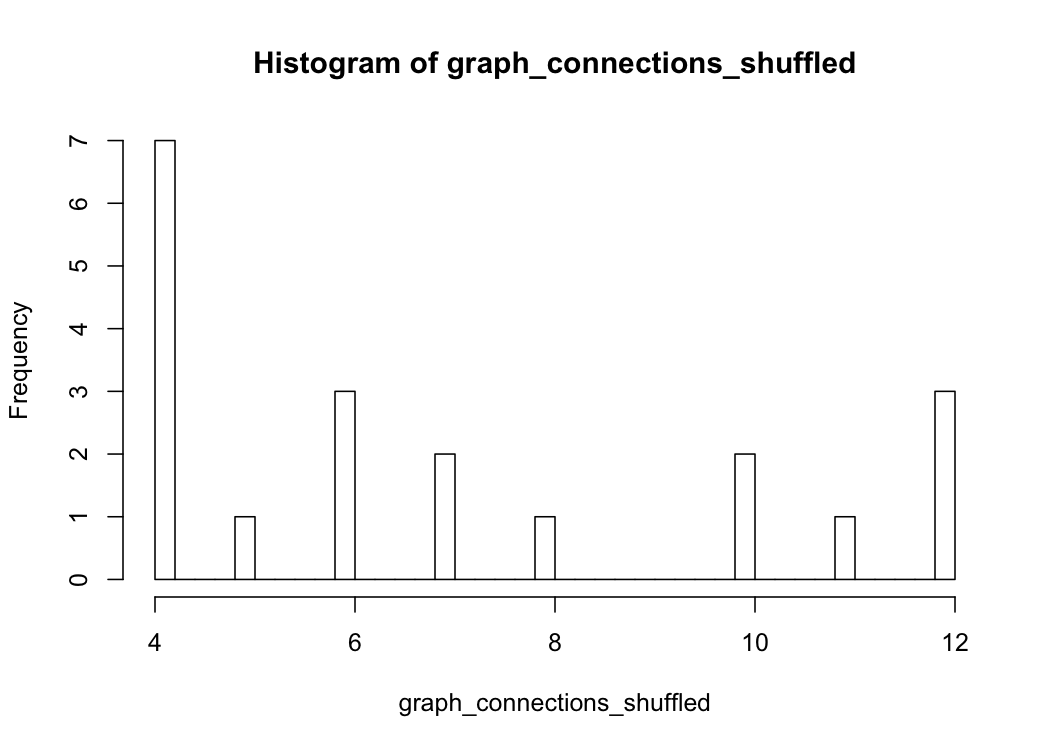The network has a distribution similar to those of biological (metabolomics) networks: Most nodes have few connections and only a few have many.

In the image of the connection matrix underlying the network, plotted below, we can see which node are connected to which.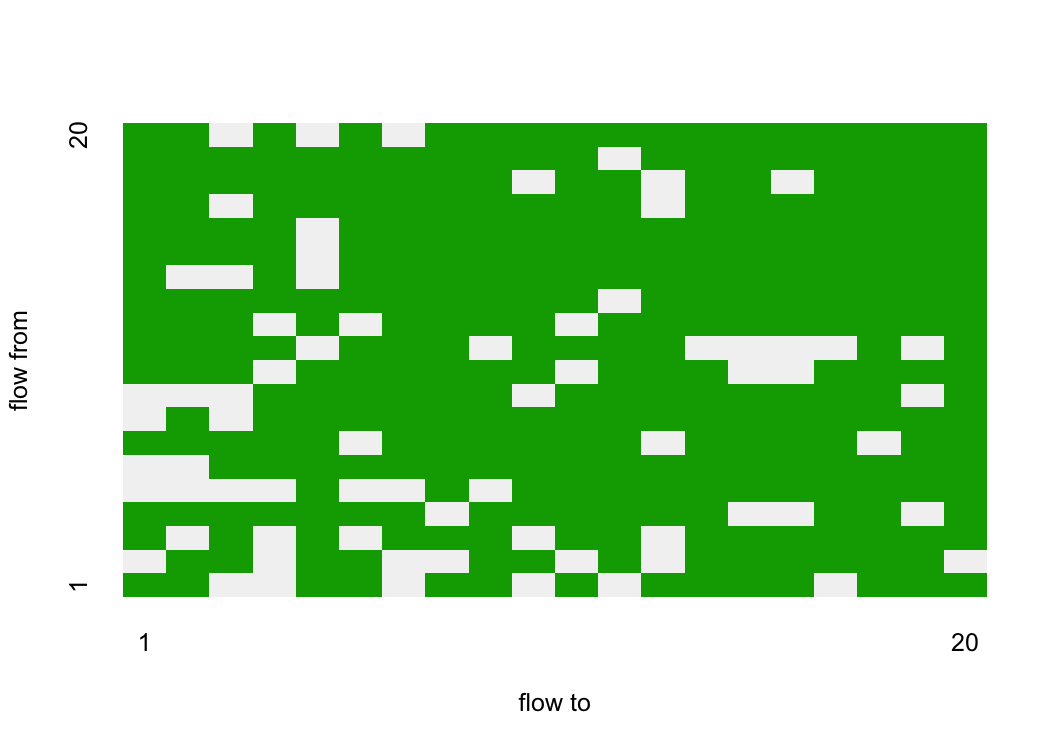### Rate initialization and mapping

All the connections indicate flow between nodes. The magnitude of the flow is governed by certain enzymes. These enzymes can be seen as the rate providing instance.

Let’s set these enzymes/rates according to a uniform distribution with values between 0 and 5 for simplicity. Next, we map these rates to the existing connections. This rate_mapping has the same dimensions as the connection matrix, however, this is not a binary matrix, instead it contains the value of the enzyme that maps to this connection.

There are a great deal more connections than enzymes, hence, a single enzyme will govern multiple connections.

### Data simulation

Now we can run a simulation. A few simulation dependent parameters need to be provided, such as time step, simulation start and end time, and a vector with initial starting concentrations for the nodes. It is also possible to provide a single starting concentrations, this initiates all nodes with the same value.

Other optional parameters can be set (see below) but we will turn these off for this first simulation.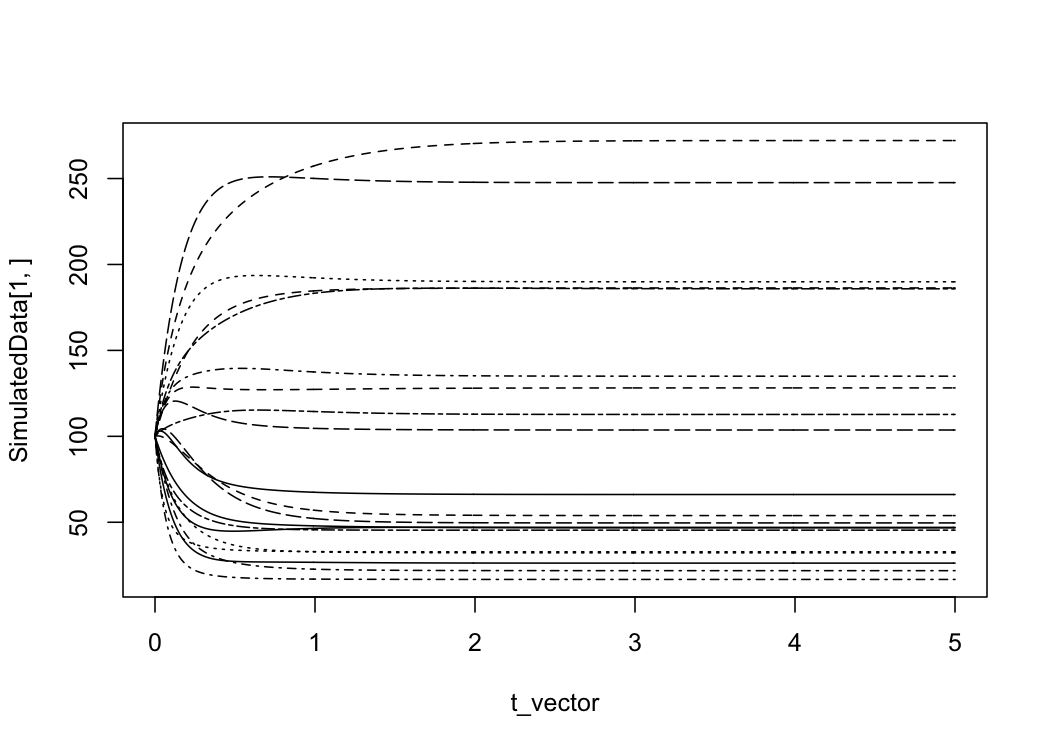Certain things can be noted. For example, the network has not reached an equilibrium state at the final time point and only a few nodes in the receive most of the concentration and most tend to 0 concentration. This is a common result when performing a simulation solely based on a network without changing rates and external influxes.

In the section below we go deeper into these aspects.

## Part 2: Additional simulation options

### Variable rates

By allowing the individual node concentrations to influence the quantity of enzymes, a new dynamic appears in the network. Let’s look at a few examples from the RateFunctionBuildR function: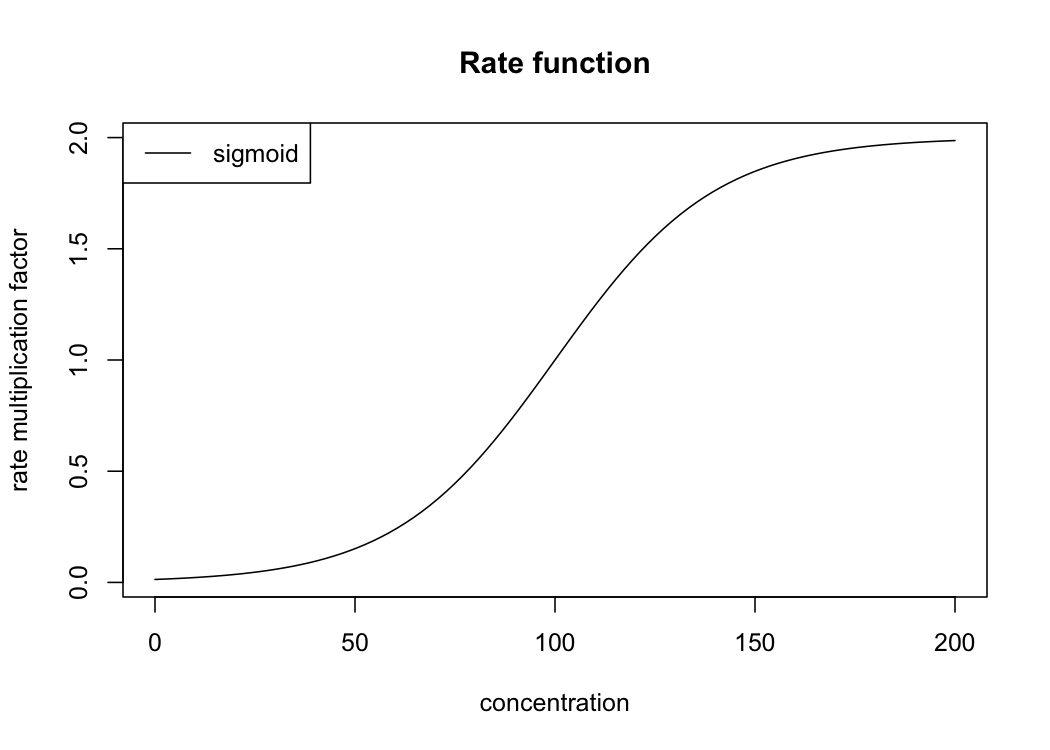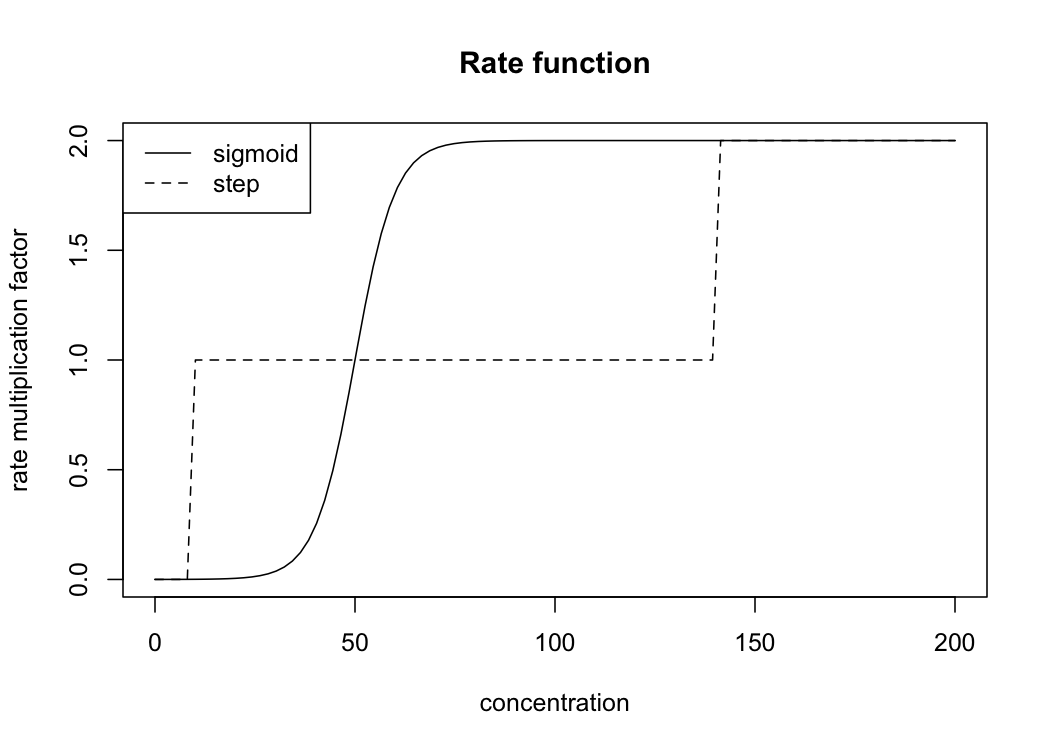The first function will result in a sigmoidal increasing factor for the rate if the source concentration rises above 100. In the second plot a comparison between a sigmoidal and stepwise rate multiplier function is illustrated. For the following simulation we will use the first sigmoidal curve (stored in the Rate_function object).

### External influxes

Next we can also include an external influx for certain nodes. This requires setting the time period of the influx as well as the vector (influx_vector) with the actual influx quantities.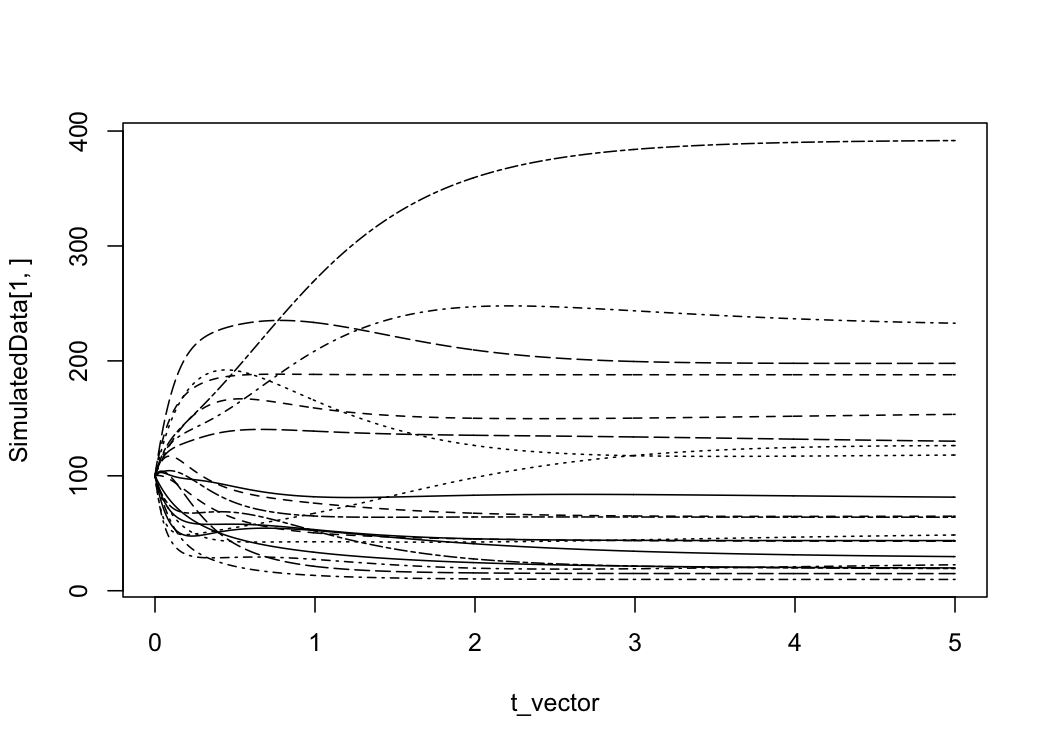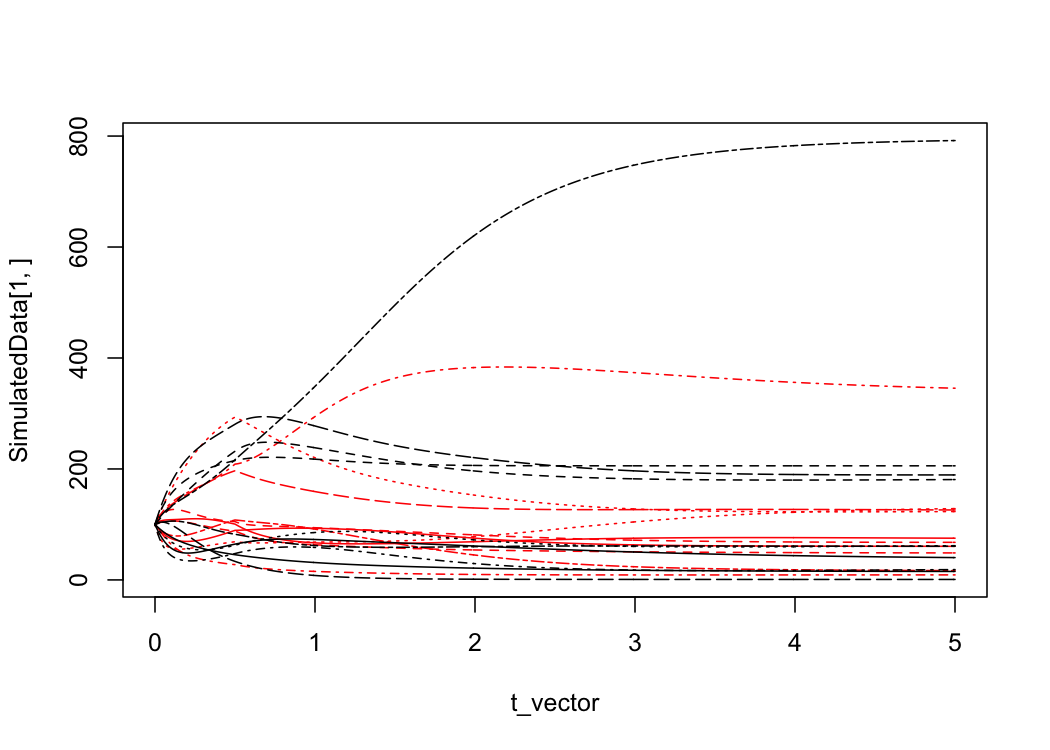We can compare the equilibrium concentration values of the network with influx vs the network without influx. For this let’s use the GetFoldChanges function which calculates the fold changes and plots the distribution.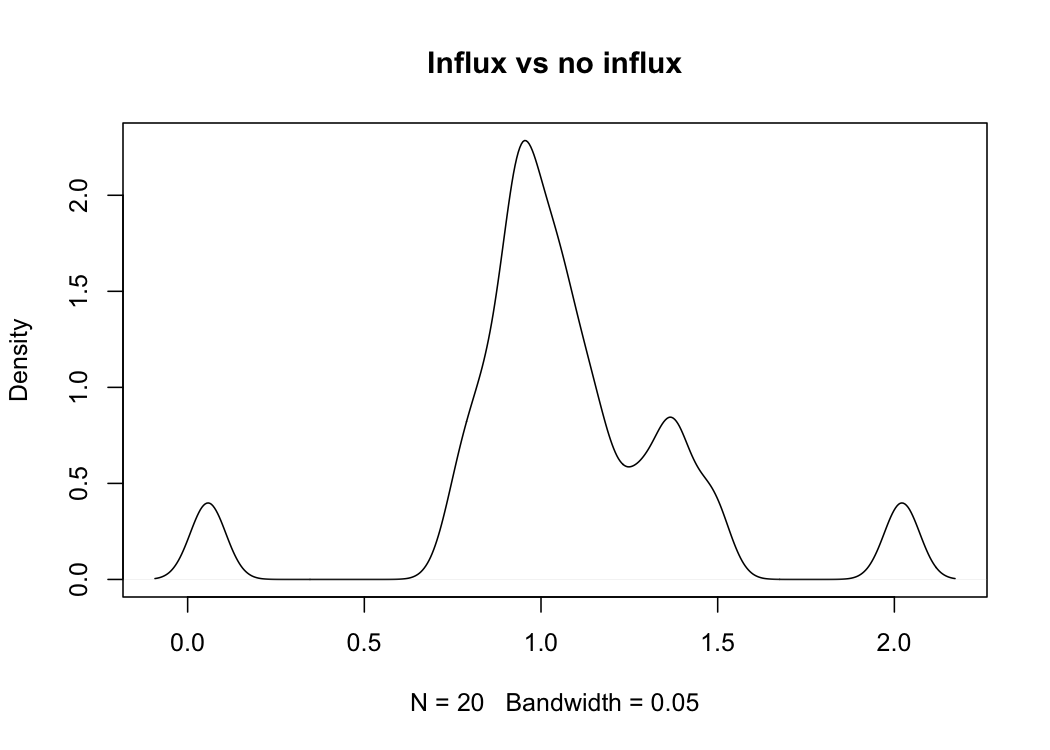Clearly, most nodes have roughly the same end state. However, some metabolites (nodes) have a substantially increased ending concentrations, whereas other nodes are almost reduced to zero in the situation with additional influx.

This draining of certain nodes is caused by the coupling of the enzymes, together with the variable rates. An influx causes an increase in concentration of node X, this causes an increase in rate x, but rate x also manages the flow from node Y to Z. Thus, depleting Y faster than in the case where no influx is present.